# Intuitive Z-plane: Part 1 – Introduction

Back in this posting, I made the following statement:

Generally speaking, digital filters work by taking an audio signal, delaying it, changing the level, and adding the result back to the signal itself.

I then showed an example of a simple digital filter like this:

If we use the filter in Figure 1, set the delay to 0, and set the gain to 1, then the output is just the input signal added to itself, so it’s two times the amplitude or about 6 dB louder.

If we leave the gain at 1, and set the delay to something else – let’s say, 1 ms, for example – then, in the very low frequencies (say, 1 Hz) the phase difference caused by the 1 ms delay is almost nothing – therefore the output will be +6 dB. At 500 Hz, however, the 1 ms delay is equal to a 180º phase shift, so the output of the delay will always be opposite in polarity with the non-delayed signal. Therefore, at 500 Hz, this filter will have no output. At 1 kHz, the output will be +6 dB again, because 1 ms = 360º at 1 kHz. At 1.5 kHz, there’s no output (540º phase shift), at 2 kHz, we’re back to +6 dB, and so on all the way up. The result is a magnitude response that looks like this: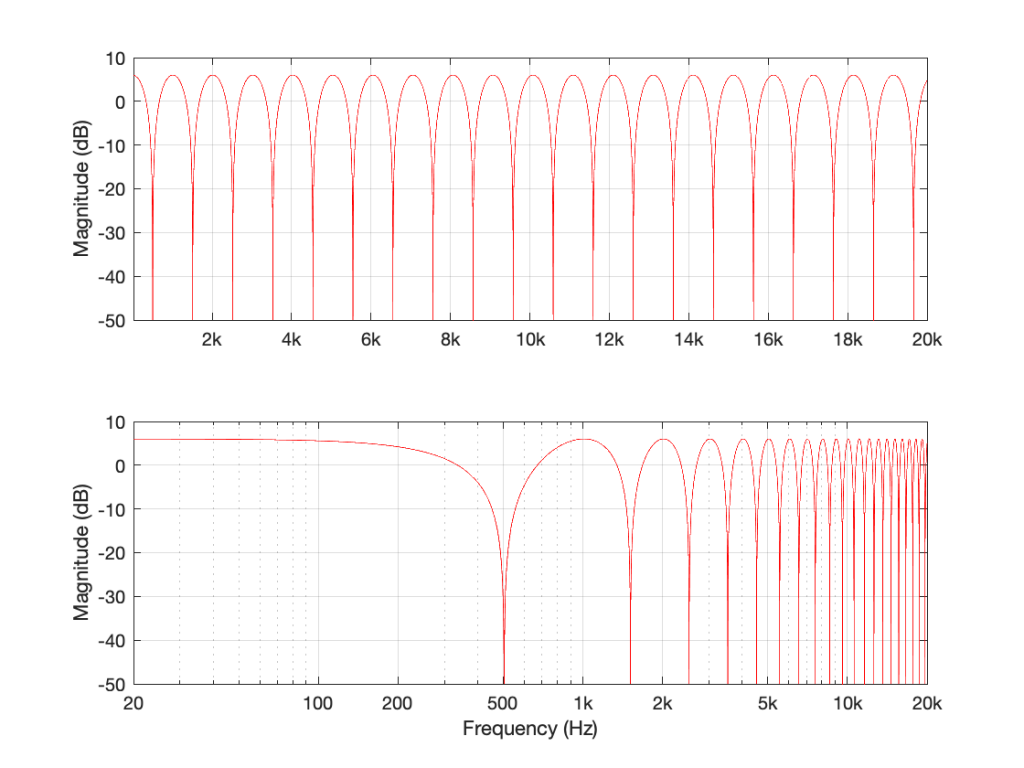Figure 2: The magnitude response of the filter shown in Figure 1, if Delay = 1 ms and Gain = 1. Note that these are two identical plots, the only difference is the scaling of the X-axes.

If I used the same delay time on the same filter structure, but set the gain to something between 0 and 1 – let’s make it 0.75, for example, then the overall shape would be the same, but the effect would be less, as shown in Figure 3.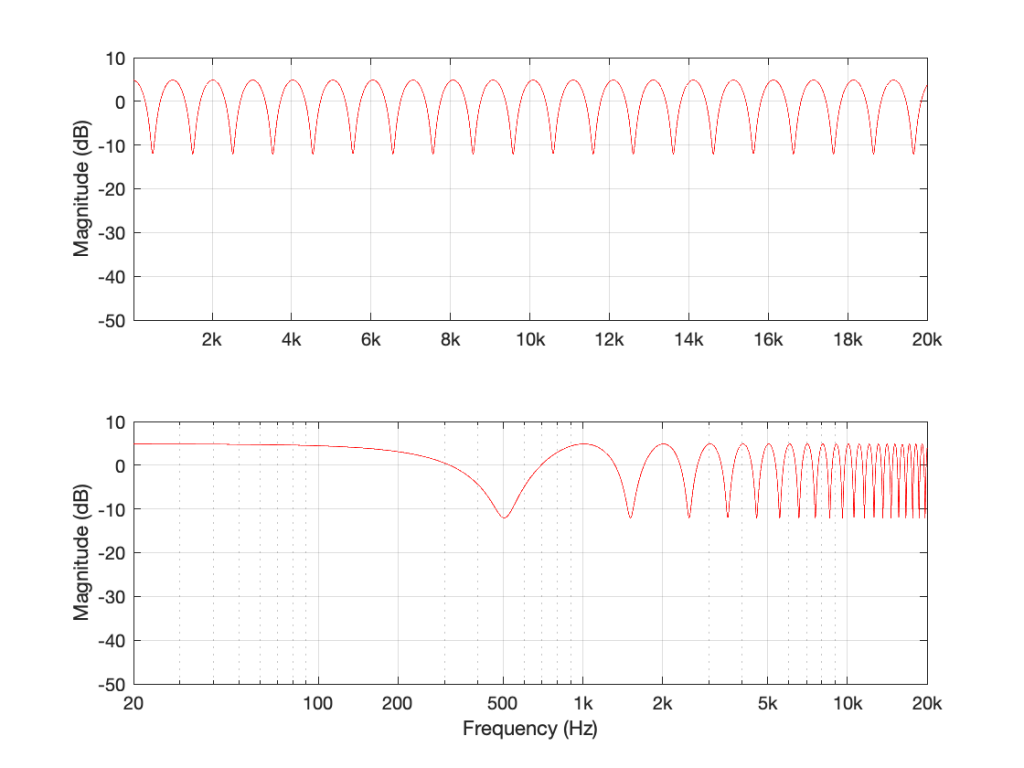Figure 3: The magnitude response of the filter shown in Figure 1, if Delay = 1 ms and Gain = 0.75.

If we make the gain a negative value, then the overall shape remains the same, but the high points and the low points swap places because the delayed signal is now cancelling where it was adding, and vice versa.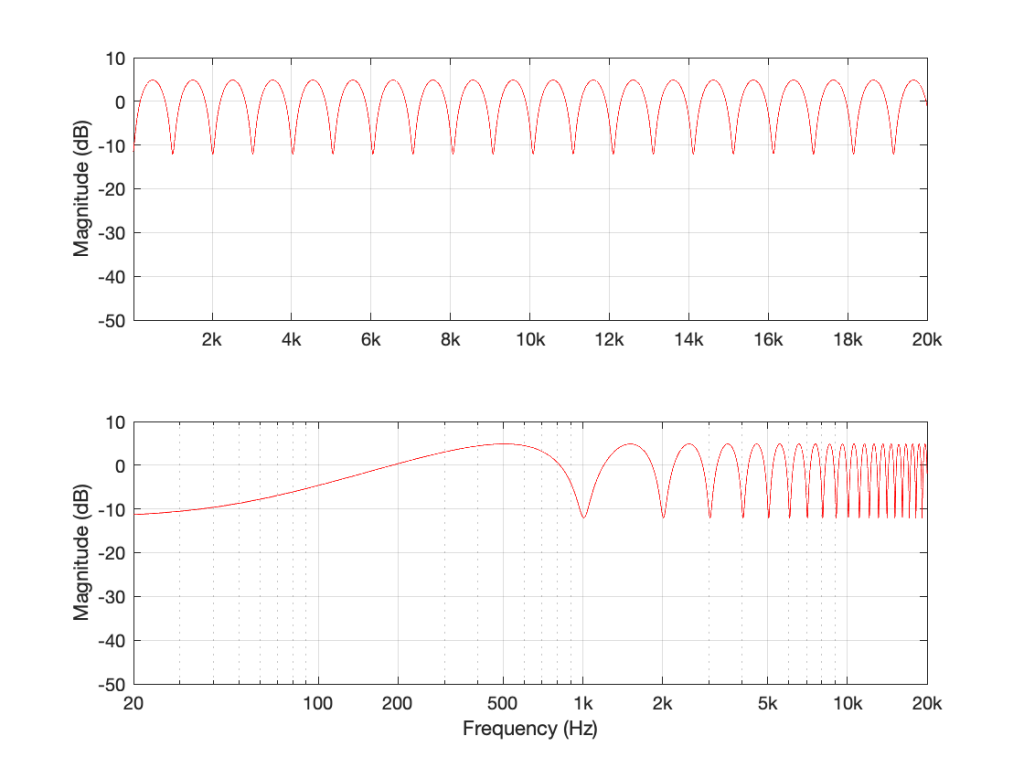Figure 4: The magnitude response of the filter shown in Figure 1, if Delay = 1 ms and Gain = -0.75. Notice how the boosts and dips have swapped places as a result of using a negative gain.

Let’s think about this intuitively. If my audio signal cannot exceed a value of 1 (which is normally the way we work… a full-scale signal ranges from -1 to 1) and the gain of the delay output in the filter in Figure 1 also ranges from -1 to 1, then the maximum possible output of the filter is 2.

If I had two delay lines and I were summing all three signals (the input and the two delayed signals) and the gains were still limited within the range of -1 to 1, then the maximum possible output would be 3…

However, the minimum possible output level (not the minimum possible output value) would be no signal (as in the case of a 500 Hz input in Figure 2. This is equivalent to an output of -∞ dB.

If I generalise this, then I can say that if your filter is built using ONLY the summed outputs of feed-forward delays, then the maximum possible output can be easily calculated, and the minimum possible output is no signal.

Still generalising: notice that the “bumps” in the above frequency responses are smooth and rounded, and the dips are pointy notches.

What happens if the filter uses feed-back instead of feed-forward?

Let’s set the delay time to 1 ms again, and set the gain to 0.99. I chose 0.99 instead of 1 because this means that each time the signal re-circulates back, it will get a little bit quieter. If I had set the gain to 1, then the delay would keep “echoing” forever. If I made it greater than 1, then the output would get louder on each re-circulation, and things would get very loud, sooner or later…

So, if Delay = 1 ms and Gain = 0.99 in the filter in Figure 5, the resulting magnitude response looks like Figure 6.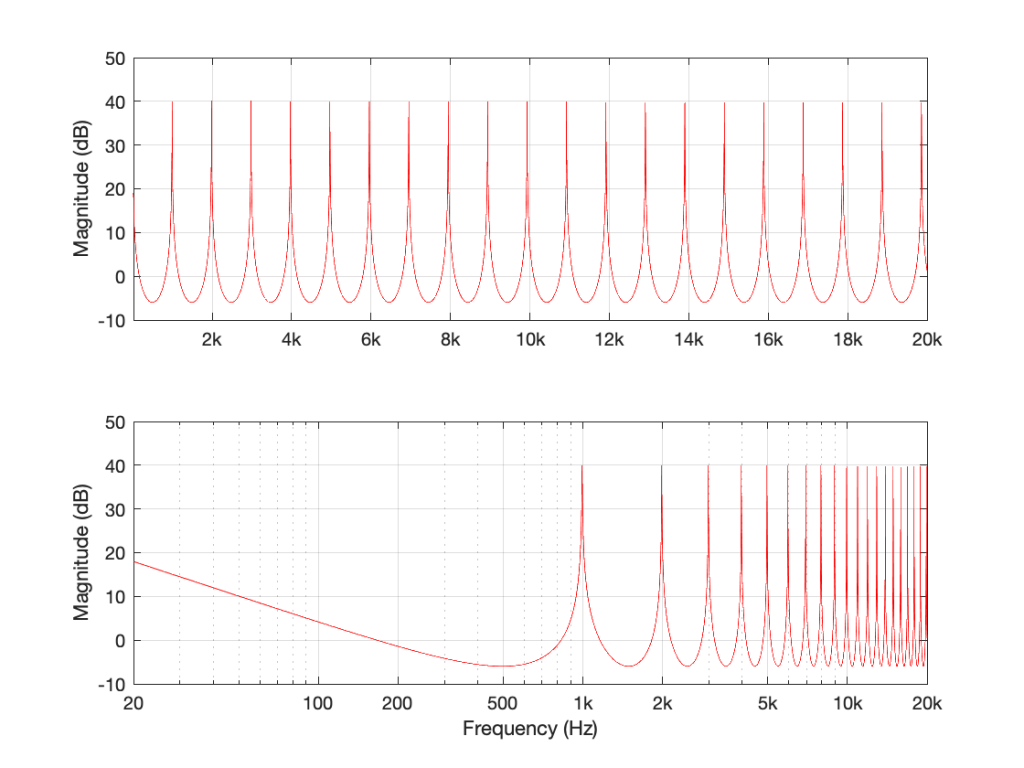Figure 6: The magnitude response of the filter in Figure 5 when Delay = 1 ms and Gain = 0.99.

There are some things to notice in Figure 6.

Firstly, notice that the overall “shape” of the curve is upside-down relative to the one in Figure 2. The rounded bits are on the bottom and the pointy bits are on the top. This means that instead of having very narrow notches, you have very narrow resonances that are “singing” like a collection of sinusoidal waves, one at the frequency of each peak.

Secondly, notice that the peaks and the dips are in the same places as in Figure 2. In both cases, the Delay = 1 ms and the gain is positive, so the frequencies that are boosted are the same in both cases. For example, both have a peak at 1 kHz, and a dip at 500 Hz.

Thirdly, notice that the overall level is much, much higher. 40 dB is a LOT louder than 6 dB – this is because the sum of all those re-circulated signals echoing over and over in the filter add up to something loud over time.

If I reduce the gain, but keep it positive, then (just like in the case with the feed-forward filter) the shape of the magnitude response stays the same, it’s just reduced in effect.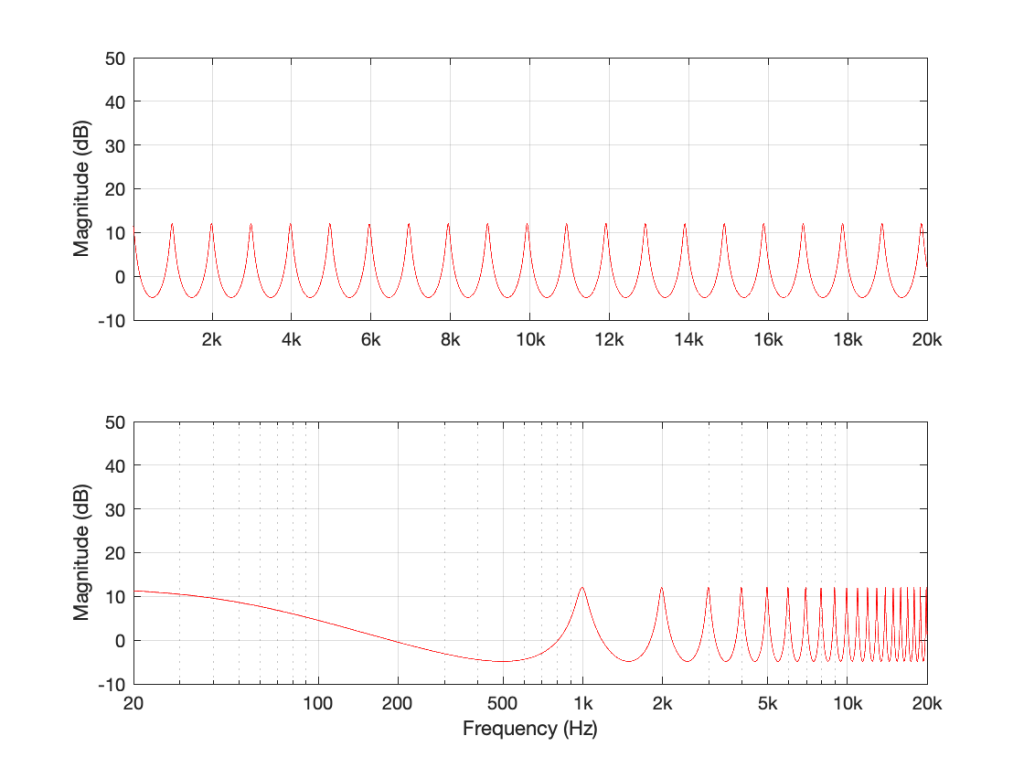Figure 7: Delay = 1 ms and Gain = 0.75. Notice that the peaks are smaller because the lower gain causes the signal to “die away” faster.

If I did the same thing, but set the gain to a negative number (say, -0.99) instead, then each time the signal re-circulates, it flips polarity. The resulting magnitude response looks like Figure 8.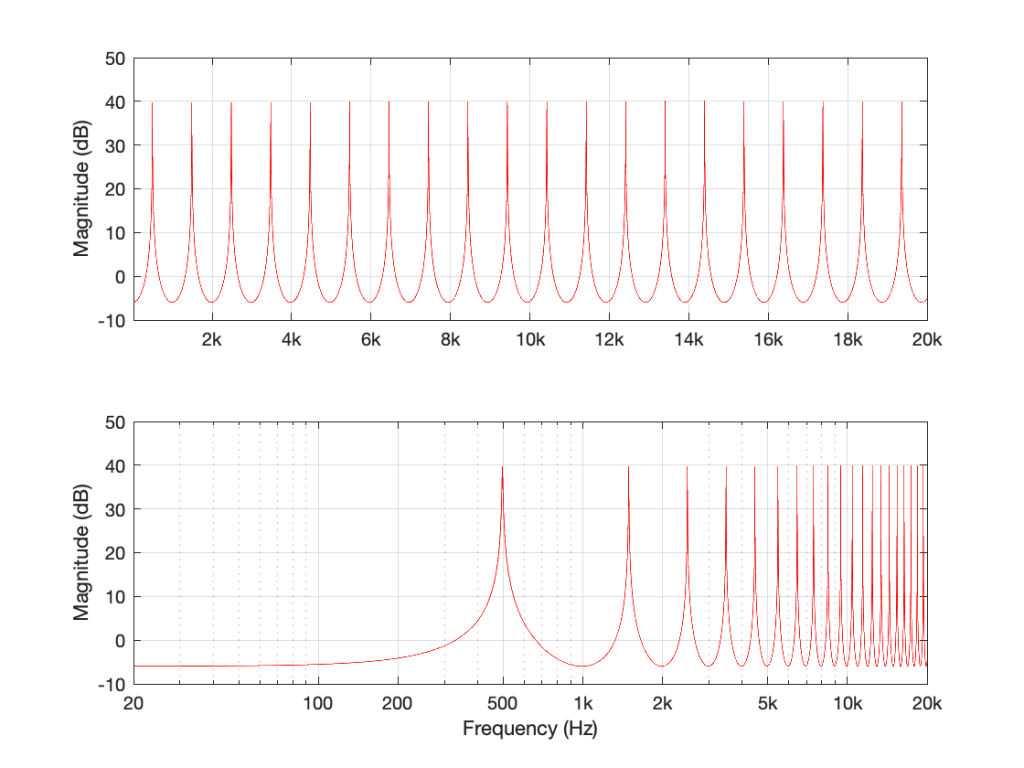Figure 8: The filter in Figure 5 where Delay = 1 ms and Gain = -0.99.

Notice that this is related to the magnitude response in Figure 4 – we have less output in the low end, and now the first peak is at 500 Hz instead of 1 kHz.

If I generalise this one, then I can say that if your filter is built using ONLY the summed outputs of feed-back delays, then the peaks are much higher than with a feed-forward design because they’re resonating.

Still generalising: notice that the “bumps” in the above frequency responses are pointy (because they’re resonances), and the dips are smooth and rounded.

## The summary (for now)

Repeating myself, because this is the take-away information for this posting:

• If your filter is built using ONLY the summed outputs of feed-forward delays, then:
• the maximum possible output can be easily calculated
• the minimum possible output is no signal
• the “bumps” in the above frequency responses are smooth and rounded
• the dips are pointy notches.
• if your filter is built using ONLY the summed outputs of feed-back delays, then:
• the peaks are much higher in level than with a feed-forward design because they’re resonating
• the “bumps” in the above frequency responses are pointy because they’re resonating
• the dips are smooth and rounded